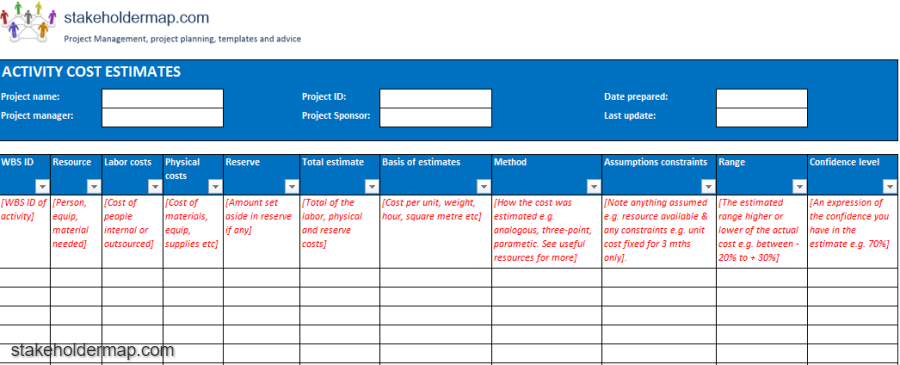# Activity Cost Estimates | Excel Template FREE

Activity Cost Estimating Template in Excel. This PMBOK template includes labor, physical and reserve costs. Basis and method of estimates, range and confidence level.

The template is fully editable with Excel and can be converted or changed to suit your project requirements. The contents of the template is shown below.## The contents of the Activity Cost Template

Document information
Enter the:
• Project name
• Project manager
• Project ID
• Date prepared
• Date of last update

WBS ID
Enter the Work Breakdown Structure (WBS) ID of the activity. This could also be an activity ID from the project schedule. The key is that it is the unique identifier for the activity that is being estimated.

procurement
The person, equipment or material that is needed for this activity. For example, 1 tonne of sand or 1 level 5 Technical Architect.

Labor costs
Enter your estimation of any labor costs.

Physical costs
Enter your estimation of any physical costs. For example the cost of 1 tonne of sand.

Reserve
If there is a reserve fund set aside enter it here.

Total estimate
This is the total of the labour, physical and reserve estimates.

Basis of estimates
Log the basis of the estimate for example, the unit cost, hourly rate, cost per square metre etc.

Method
Note how the cost was estimated for example analogous, parametric, three-point estimation, expert opinion etc.

### Get a duration estimation template using Analogous, Parametric and Three-point estimation.

Assumptions constraints
Document whether anything was assumed to be true when making the estimate for example, that resources would be available or that unit price would remain fixed.

Range
Here show the expected range in which the actual costs could fall. For example, actual costs maybe between 20 percent lower to 30 percent higher than the estimate.

Confidence level
Give the level of confidence that you have in the estimate. For example, 70 percent confidence.